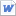#검색어 입력폼# 랜덤 변수 발생 ,중심극한정리

저작시기 2009.06 |등록일 2009.06.08 | 최종수정일 2015.09.18MS 워드 (docx) | 8페이지 | 가격 10,000원

## 소개글

기각법, 중심극한정리, 가우시안 랜덤 변수,
generating random vraibale using transformation method, rejection method,
central limit theorm, 에관련된 자료 입니다.

## 목차

1) The Inverse Transform Algorithm.
2) The Rejection Method
Reference

## 본문내용

1) The Inverse Transform Algorithm.
Proposition
Let U be a uniform (0,1) random variable. For any continuous distribution function F the random variable X defined by X=Inv (F (U)) has distribution F.
Proof
Let Fx denote the distribution function of X=Inv (F (U)). Then,
Fx(x) =P{X<=x}
=P {Inv (F (U)) <=x}
Now since F is a distribution function it follows that F(x) is a monotone increasing function of x and so the inequality “a<=b” is equivalent to the inequality “F (a)<=F(b)”.
Hence, we see that
Fx(x)=P{F(inv(F(U)))<=F(x)}
=P {U<=F(x)} (since F(inv(F(U))=U)
=F(x) (since U is uniform (0, 1))

Implementation by using MATLAB

n=10000;
uni=rand(1,n);
X=sqrt(uni)
[N,h]=hist(X,100)
N=N/(h(2)-h(1))./n;
bar(h,N,1,`w`)
hold on

x=0:1;
y=2*x
plot(x,y);

## 참고 자료

1. Computational statistics handbook, with Matlab, J.E. Gentle Wolfgang Hsrdle
2. Simulation, second edition, Sheldon M.Ross.
3. Main textbook of our class.